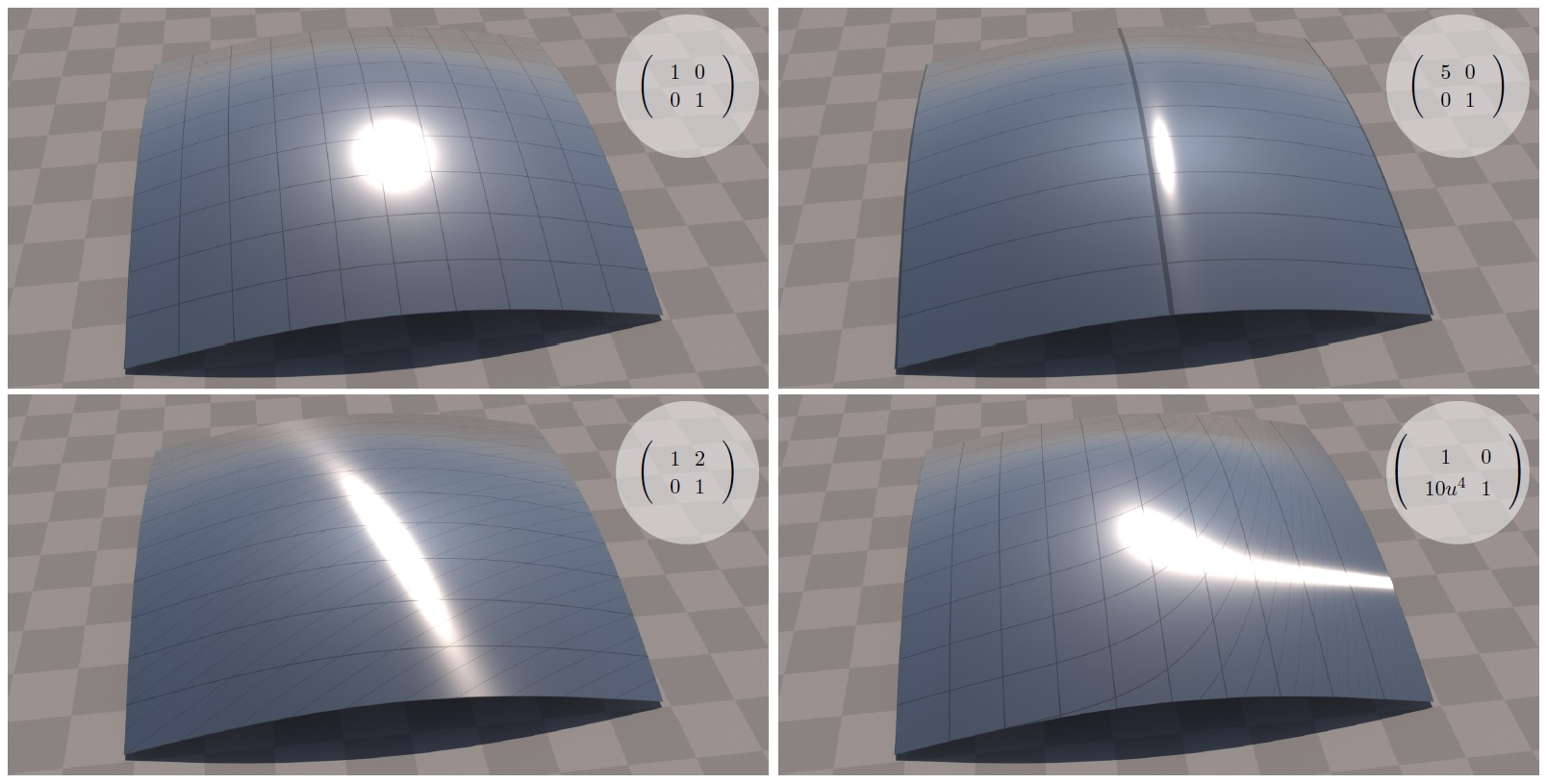A demonstration how different 2D transformations (top right insets) of the microsurface affect the reflection off a rough silver plate. Top left: The original unmodified surface with shape-variant GTR distribution with tail γ = 1.7 and roughness α = 0.0121. A 10×10 grid covers the unit texture space to serve as a reference. Top right: The microsurface is stretched 5 times horizontally. This makes the surface smoother in that direction, so the highlight is compressed accordingly. Bottom left: A skew transform. Bottom right: An example of a non-linear transformation: T(u, v) = (u, v+2u5). The Jacobian matrix JT (top right inset) is a local linear approximation of T and we apply it as a local tangential transform. Notice that the prolonged and curved shape of the highlight to the right is due to more surface compression in that region.

# Microsurface Transformations

## Abstract

We derive a general result in microfacet theory: given an arbitrary microsurface defined via standard microfacet statistics, we show how to construct the statistics of its linearly transformed counterparts. A common use case of such transformations is to generate anisotropic versions of a given surface. Traditional anisotropic derivations based on varying the roughness of an isotropic distribution in an ellipse have a general closed-form formula only for the subclass of shape-invariant distributions. While our formulation is equivalent to these specific constructs, it is more general in two aspects: it leads to simple closed-form solutions for all distributions, including shape-variant ones, and works for all invertible 2D transform matrices. The latter is of particular importance in case of deformation of the macrosurface, since it can be approximated locally by a linear transformation in the tangent plane. We demonstrate our results using the Generalized Trowbridge-Reitz (GTR) distribution which is shape-invariant only in the special case of the popular Trowbridge-Reitz (GGX) distribution.

Play Video

## BibTex Citation

```				```
@article{https://doi.org/10.1111/cgf.14590,

author = {Atanasov, A. and Koylazov, V. and Dimov, R. and Wilkie, A.},

title = {Microsurface Transformations},

journal = {Computer Graphics Forum},

volume = {41},

number = {4},

pages = {105-116},

keywords = {CCS Concepts, • Computing methodologies → Rendering, Reflectance modeling},

doi = {https://doi.org/10.1111/cgf.14590},

url = {https://onlinelibrary.wiley.com/doi/abs/10.1111/cgf.14590},

eprint = {https://onlinelibrary.wiley.com/doi/pdf/10.1111/cgf.14590},

abstract = {Abstract We derive a general result in microfacet theory: given an arbitrary microsurface defined via standard microfacet statistics, we show how to construct the statistics of its linearly transformed counterparts. A common use case of such transformations is to generate anisotropic versions of a given surface. Traditional anisotropic derivations based on varying the roughness of an isotropic distribution in an ellipse have a general closed-form formula only for the subclass of shape-invariant distributions. While our formulation is equivalent to these specific constructs, it is more general in two aspects: it leads to simple closed-form solutions for all distributions, including shape-variant ones, and works for all invertible 2D transform matrices. The latter is of particular importance in case of deformation of the macrosurface, since it can be approximated locally by a linear transformation in the tangent plane. We demonstrate our results using the Generalized Trowbridge-Reitz (GTR) distribution which is shape-invariant only in the special case of the popular Trowbridge-Reitz (GGX) distribution.},

year = {2022}

}
```
```